# 11.  The probability that the coin lands tails is 40%. Because the probability is not...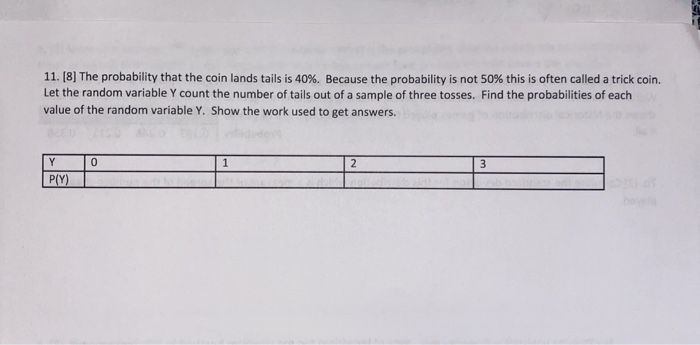11.  The probability that the coin lands tails is 40%. Because the probability is not 50% this is often called a trick coin. Let the random variable y count the number of tails out of a sample of three tosses. Find the probabilities of each value of the random variable Y. Show the work used to get answers. 0 1 2 3 Y PY)

Ans 1)

Probability of success (drawing a tail) = 40/100 = 0.4

Total number of trials n = 3

Formula to calculate Binomial probability: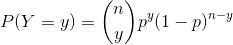Probability when there is 0 tail: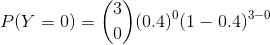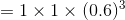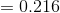---------------------------

Probability when there is 1 tail: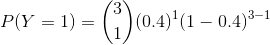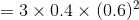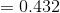---------------------------------------

Probability when there is 2 tail: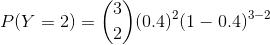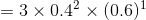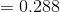----------------------------------------

Probability when there is 3 tail: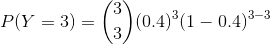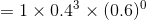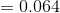Y 0 1 2 3 P(Y) 0.216 0.432 0.288 0.064

#### Earn Coin

Coins can be redeemed for fabulous gifts.

Similar Homework Help Questions
• ### Let W be a random variable giving the number of heads minus the number of tails in three tosses of a coin

3. Let W be a random variable giving the number of heads minus the number of tails in three tosses of a coin. List the elements of the sample space S for the three tosses of the coin and to each sample point assign a value w of W.

• ### Assume that a coin is flipped where the probability of coin lands "Heads" is 0.49. The...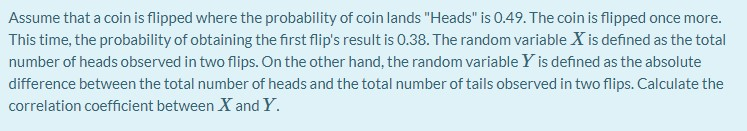Assume that a coin is flipped where the probability of coin lands "Heads" is 0.49. The coin is flipped once more. This time, the probability of obtaining the first flip's result is 0.38. The random variable X is defined as the total number of heads observed in two flips. On the other hand, the random variable Y is defined as the absolute difference between the total number of heads and the total number of tails observed in two flips. Calculate...

• ### You toss a penny and observe whether it lands heads up or tails up. Suppose the...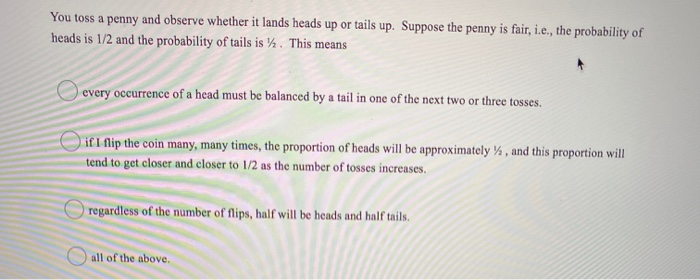You toss a penny and observe whether it lands heads up or tails up. Suppose the penny is fair, i.e., the probability of heads is 1/2 and the probability of tails is y. This means every occurrence of a head must be balanced by a tail in one of the next two or three tosses. if I flip the coin many, many times, the proportion of heads will be approximately %, and this proportion will tend to get closer and...

• ### 3. (PMF – 8 points) Consider a sequence of independent trials of fair coin tossing. Let...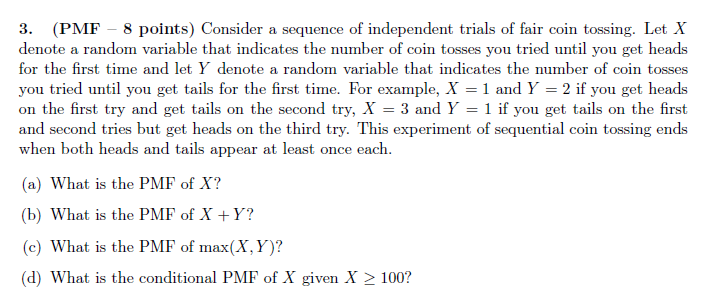3. (PMF – 8 points) Consider a sequence of independent trials of fair coin tossing. Let X denote a random variable that indicates the number of coin tosses you tried until you get heads for the first time and let y denote a random variable that indicates the number of coin tosses you tried until you get tails for the first time. For example, X = 1 and Y = 2 if you get heads on the first try and...

• ### All work must be shown 20. An unfair coin has probability 0.65 of landing tails. The...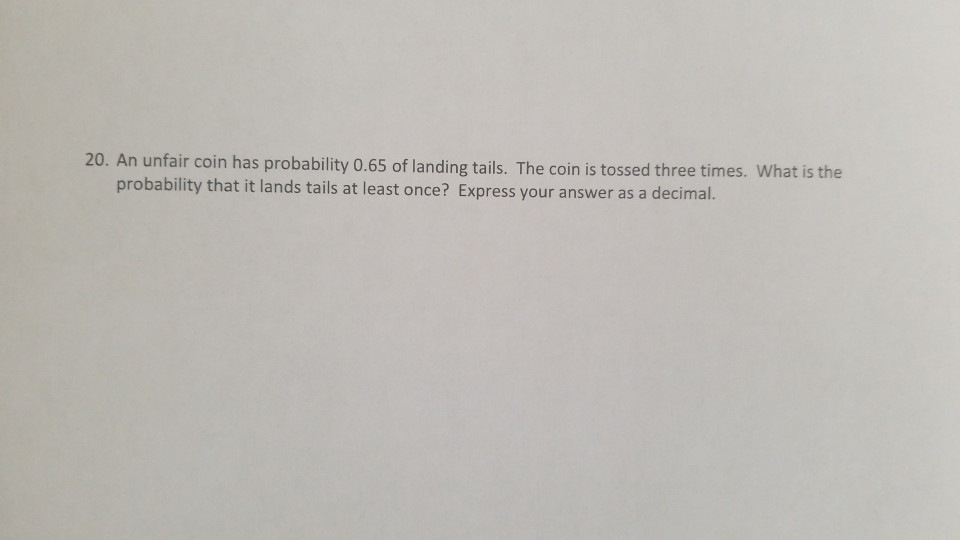All work must be shown 20. An unfair coin has probability 0.65 of landing tails. The coin is tossed three times. What is the probability that it lands tails at least once? Express your answer as a decimal.

• ### Question 2 (2 points) Suppose Tori has an unfair coin which lands on Tails with probability...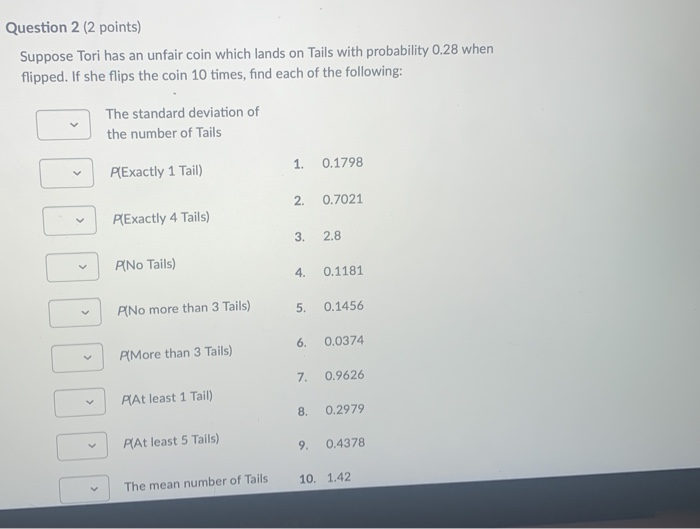Question 2 (2 points) Suppose Tori has an unfair coin which lands on Tails with probability 0.28 when flipped. If she flips the coin 10 times, find each of the following: The standard deviation of the number of Tails PExactly 1 Tail) 1. 0.1798 2. 0.7021 PExactly 4 Tails) 3. 2.8 PiNo Tails) 4 0.1181 P[No more than 3 Tails) 5. 0.1456 PlMore than 3 Tails) 6. 0.0374 7. 0.9626 PlAt least 1 Tail) 8. 0.2979 PAt least 5 Tails)...

• ### 4. Suppose urn 11 is filled with 60% green balls and 40% red balls, and urn...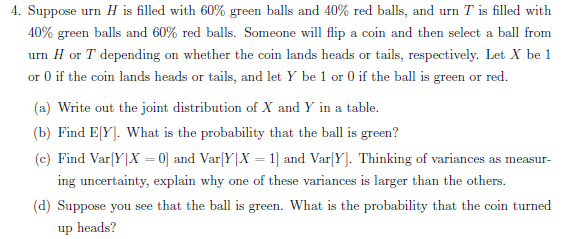4. Suppose urn 11 is filled with 60% green balls and 40% red balls, and urn T is filled with 40% green balls and 60% red balls. Someone will flip a coin and then select a ball from urn H or T depending on whether the coin lands heads or tails, respectively. Let X be 1 or 0 if the coin lands heads or tails, and let Y be 1 or 0 if the ball is green or red (a)...

• ### A biased coin is tossed n times. The probability of heads is p and the probability of tails is q and p=2q. Choose all correct statements. This is an example of a Bernoulli trial n-n-1-1-(k-1) p...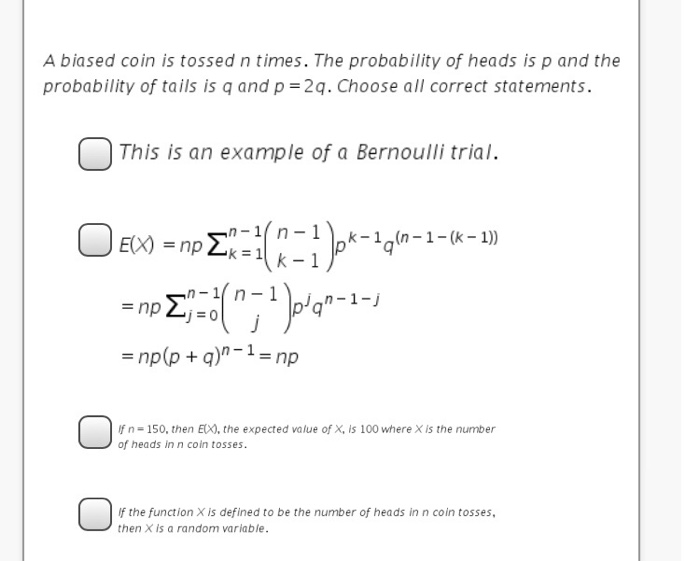A biased coin is tossed n times. The probability of heads is p and the probability of tails is q and p=2q. Choose all correct statements. This is an example of a Bernoulli trial n-n-1-1-(k-1) p'q =np(p + q)n-1 = np f n- 150, then EX), the expected value of X, is 100 where X is the number of heads in n coin tosses. f the function X is defined to be the number of heads in n coin tosses,...

• ### 2. SUPPLEMENTAL QUESTION 1 (a) Toss a fair coin so that with probability pheads occurs and...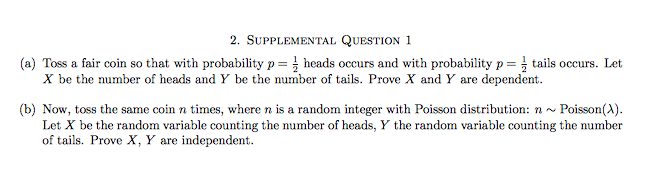2. SUPPLEMENTAL QUESTION 1 (a) Toss a fair coin so that with probability pheads occurs and with probability p tails occurs. Let X be the number of heads and Y be the number of tails. Prove X and Y are dependent (b) Now, toss the same coin n times, where n is a random integer with Poisson distribution: n~Poisson(A) Let X be the random variable counting the number of heads, Y the random variable counting the number of tails. Prove...

• ### 1. Consider flipping a fair coin three times and observe whether it lands heads up or...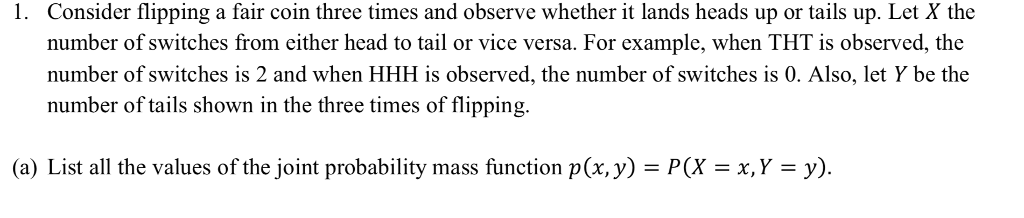1. Consider flipping a fair coin three times and observe whether it lands heads up or tails up. Let X the number of switches from either head to tail or vice versa. For example, when THT is observed, the number of switches is 2 and when HHH is observed, the number of switches is 0. Also, let Y be the number of tails shown in the three times of fipping. (a) List all the values of the joint probability mass...